# Frank Solutions for Class 10 Maths Chapter 4 Shares and Dividends

Frank Solutions for Class 10 Maths Chapter 4 Shares and Dividends are available in PDF here. These Solutions will help students to clear their doubts quickly and also assist in learning the topic effectively. Students can refer to these solutions whenever they face difficulty while answering the textbook questions. Students of Class 10 are suggested to practise Frank Solutions for Class 10 Maths to strengthen their fundamentals and be able to solve questions that are usually asked in the board examination.

In Chapter 4, Shares and Dividends, the dividend is defined as a payment made by a corporation to its shareholders. Usually, these payments are made in cash, but sometimes companies will also distribute stock dividends, whereby additional stock shares are distributed to shareholders. This chapter contains topics related to dividends, shares and investments, etc.

## Download the PDF of Frank Solutions for Class 10 Maths Chapter 4 Shares and Dividends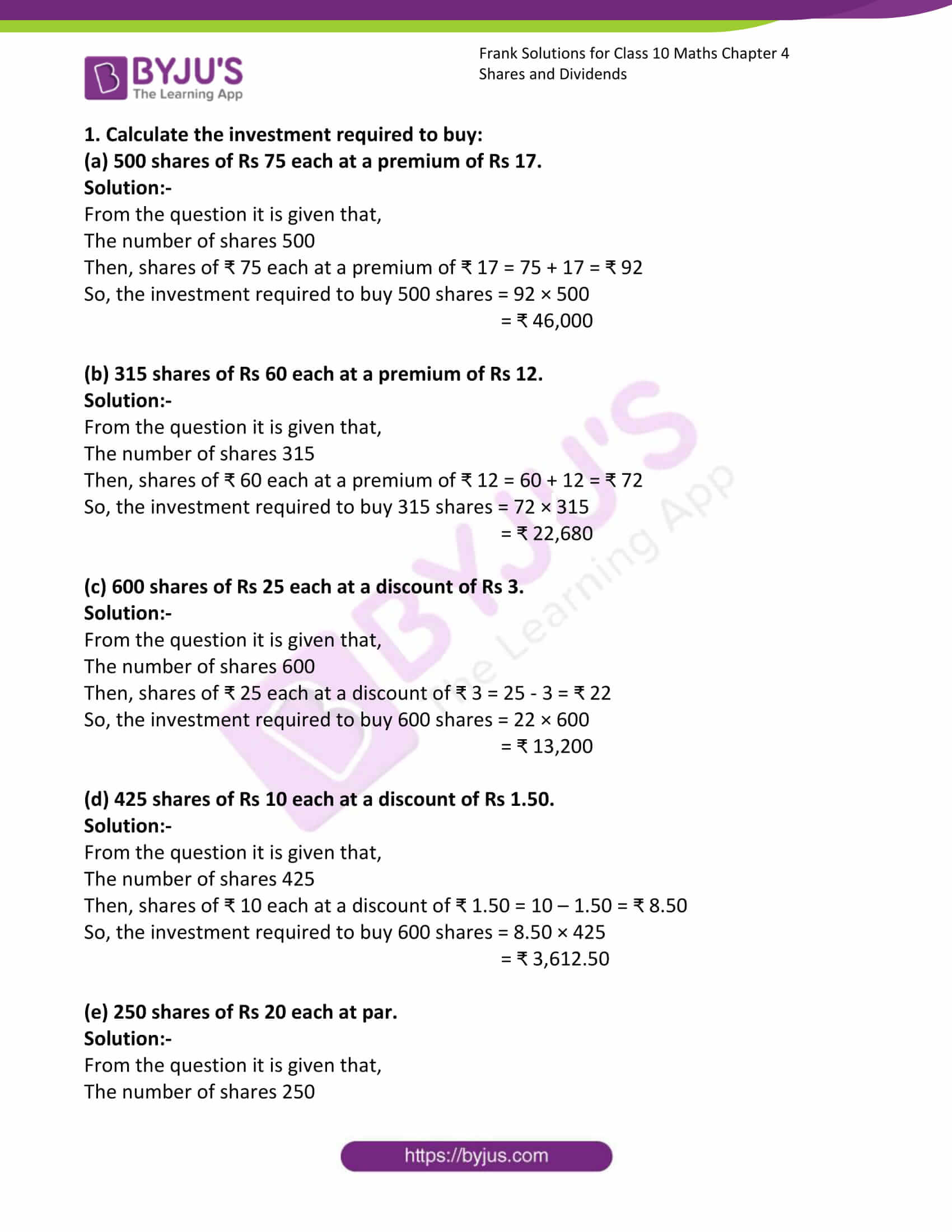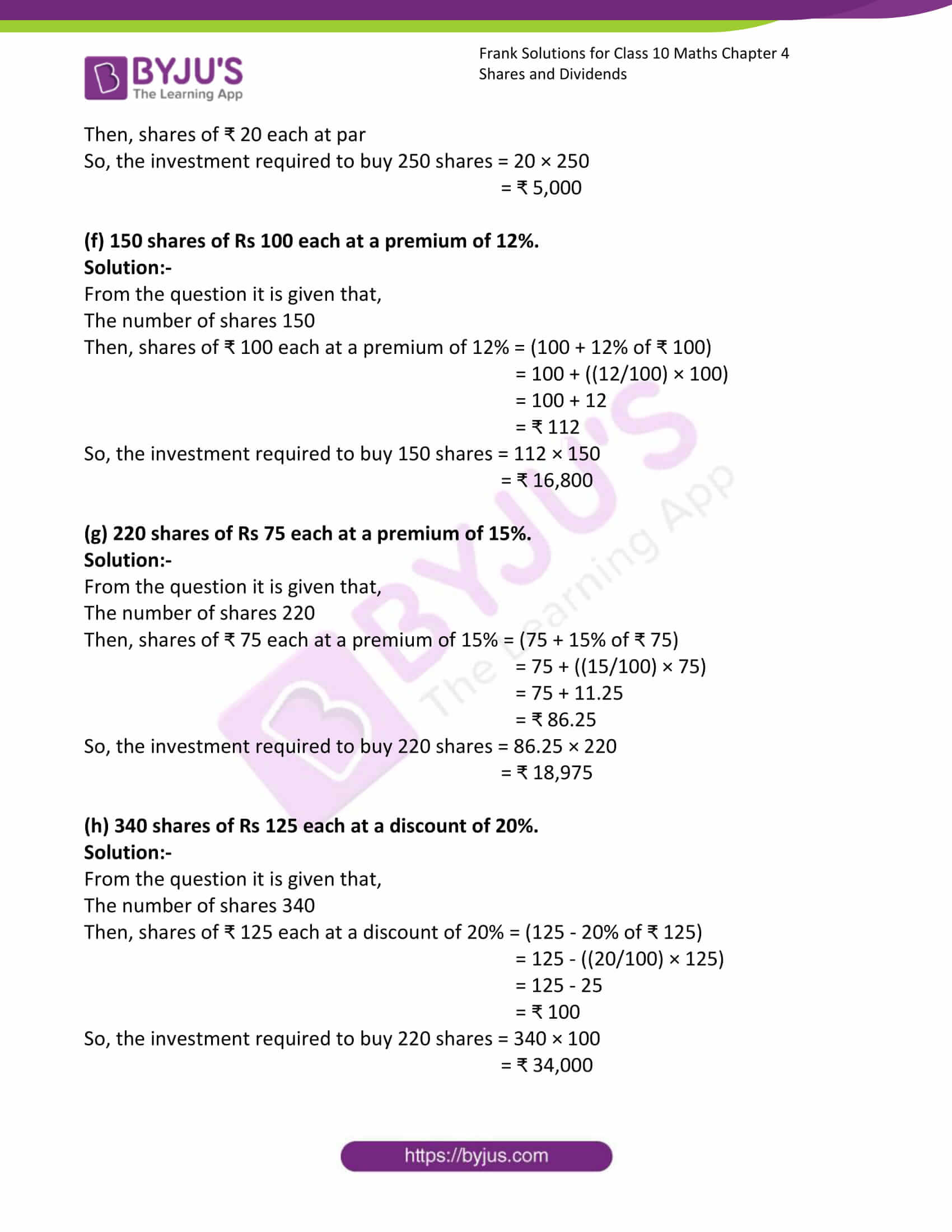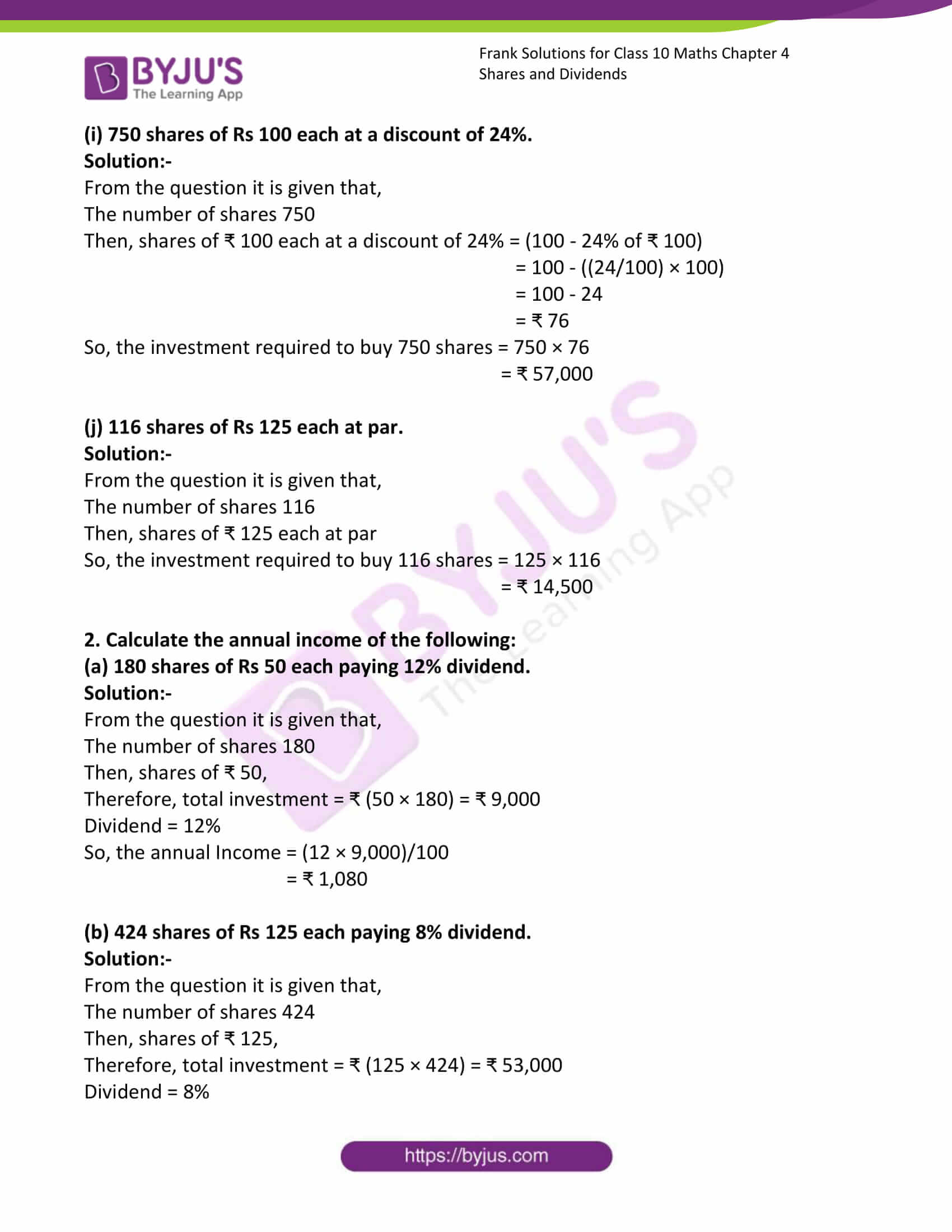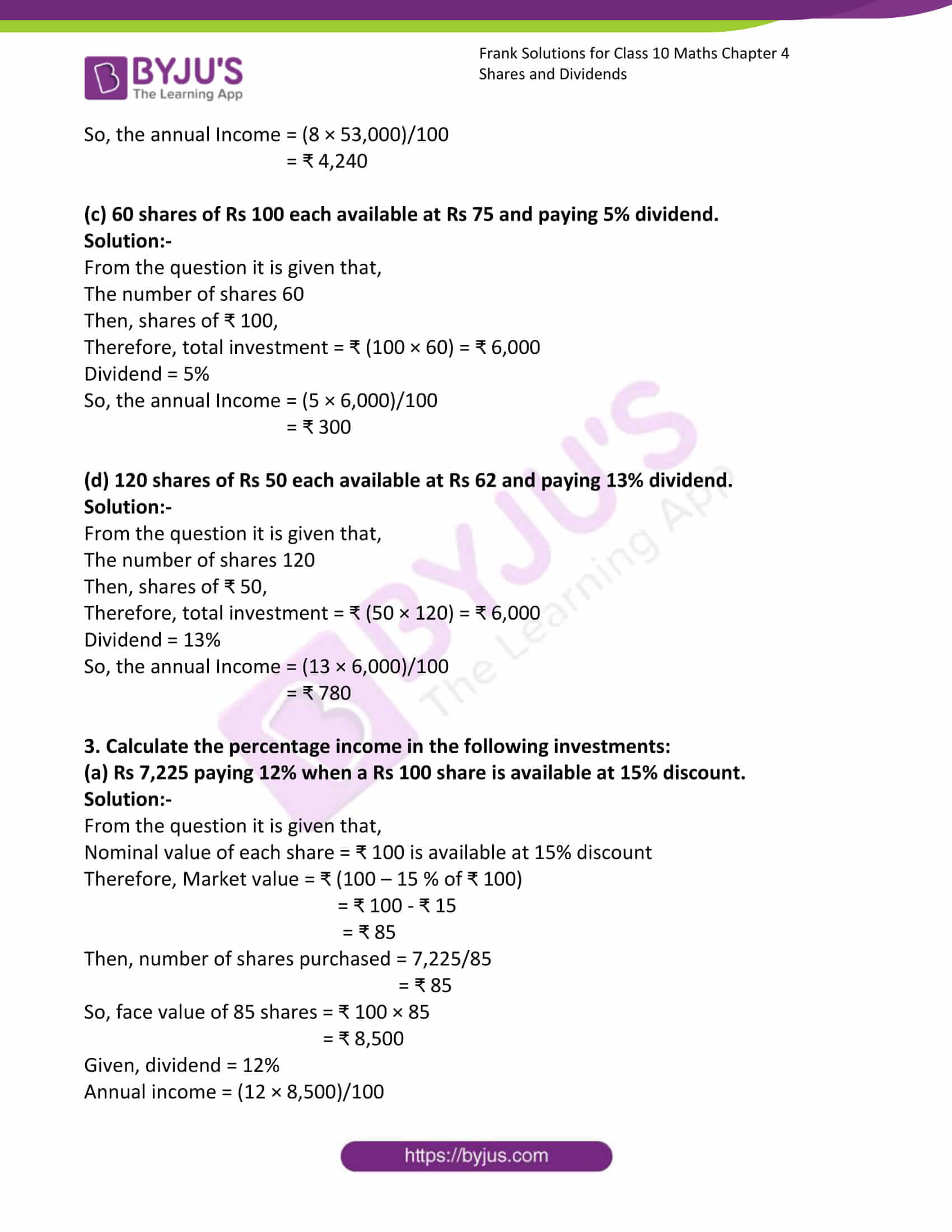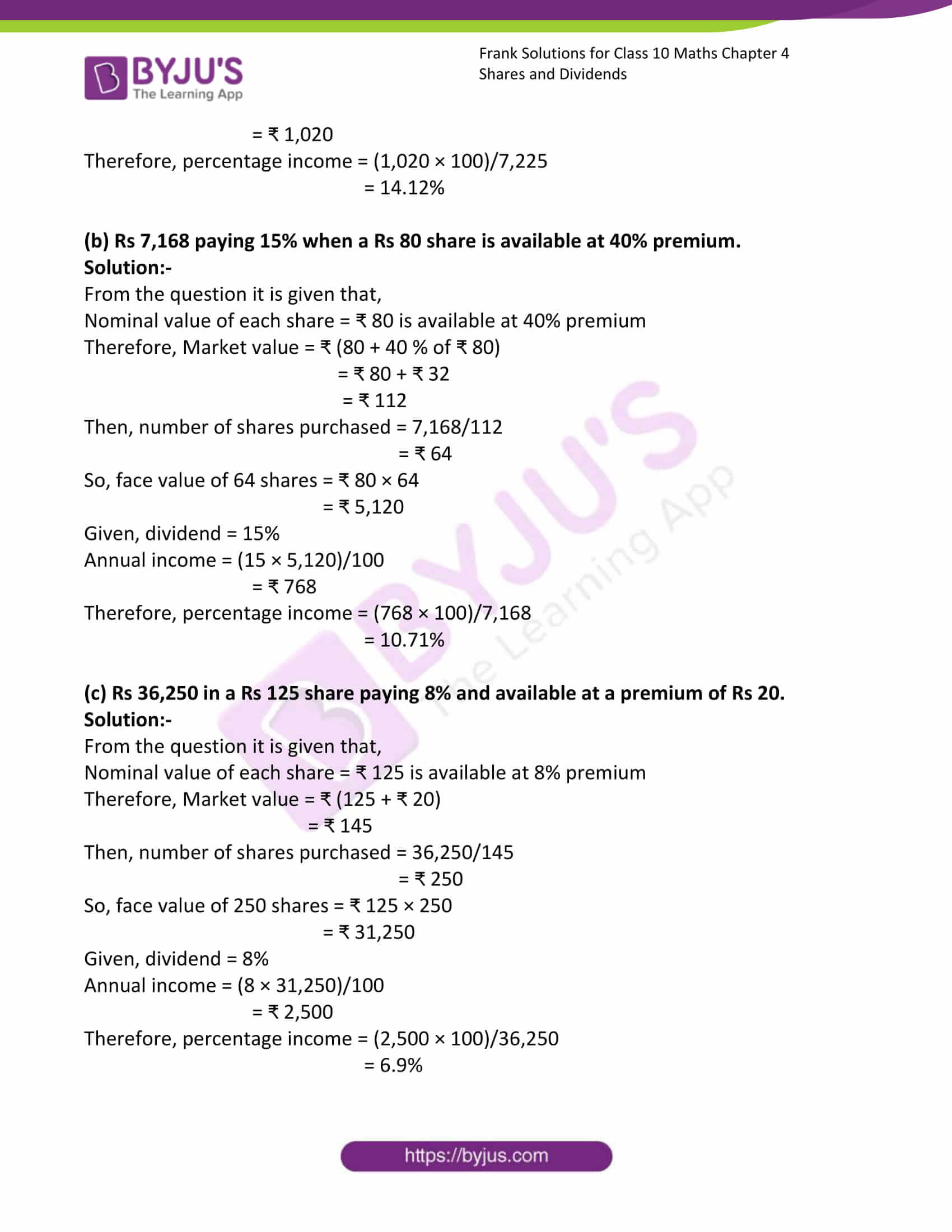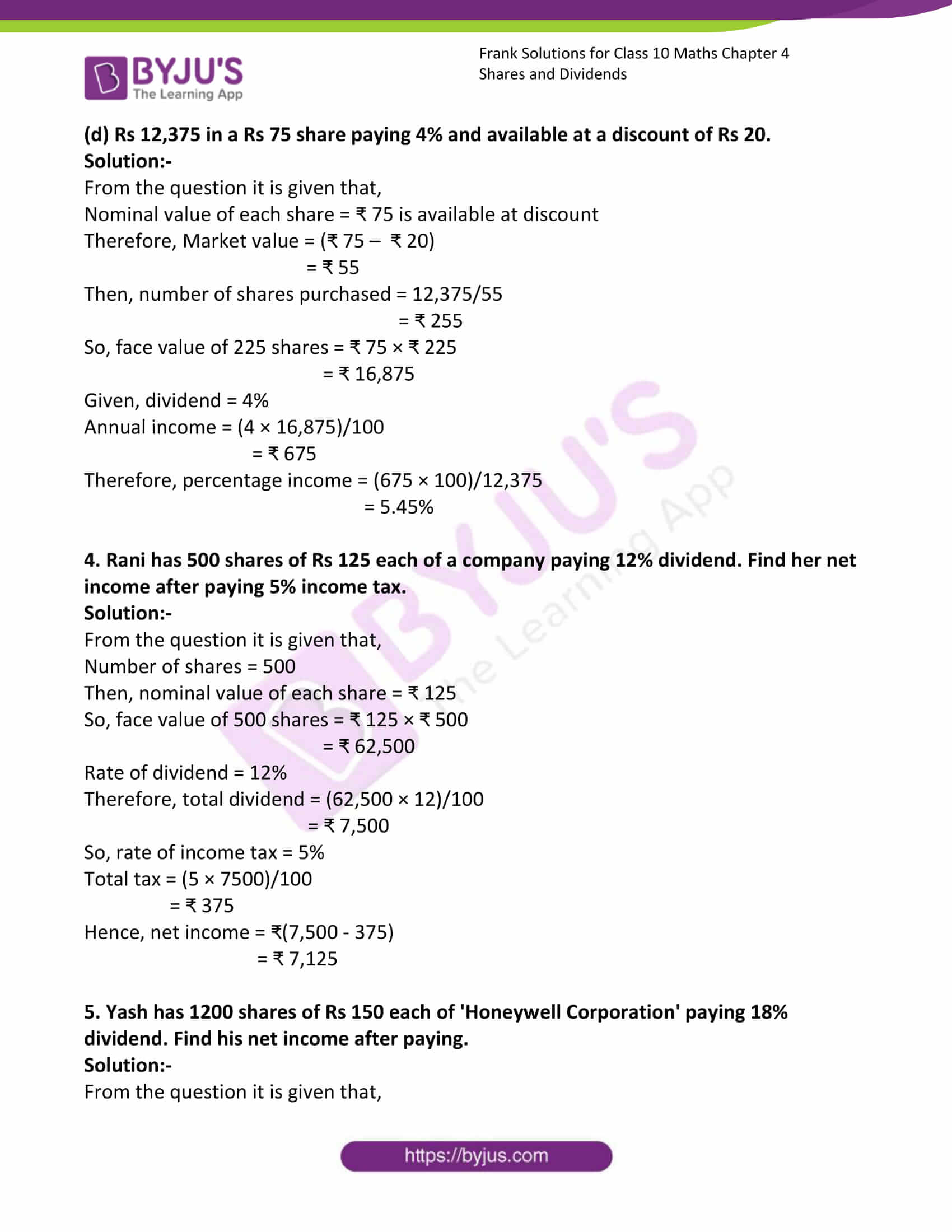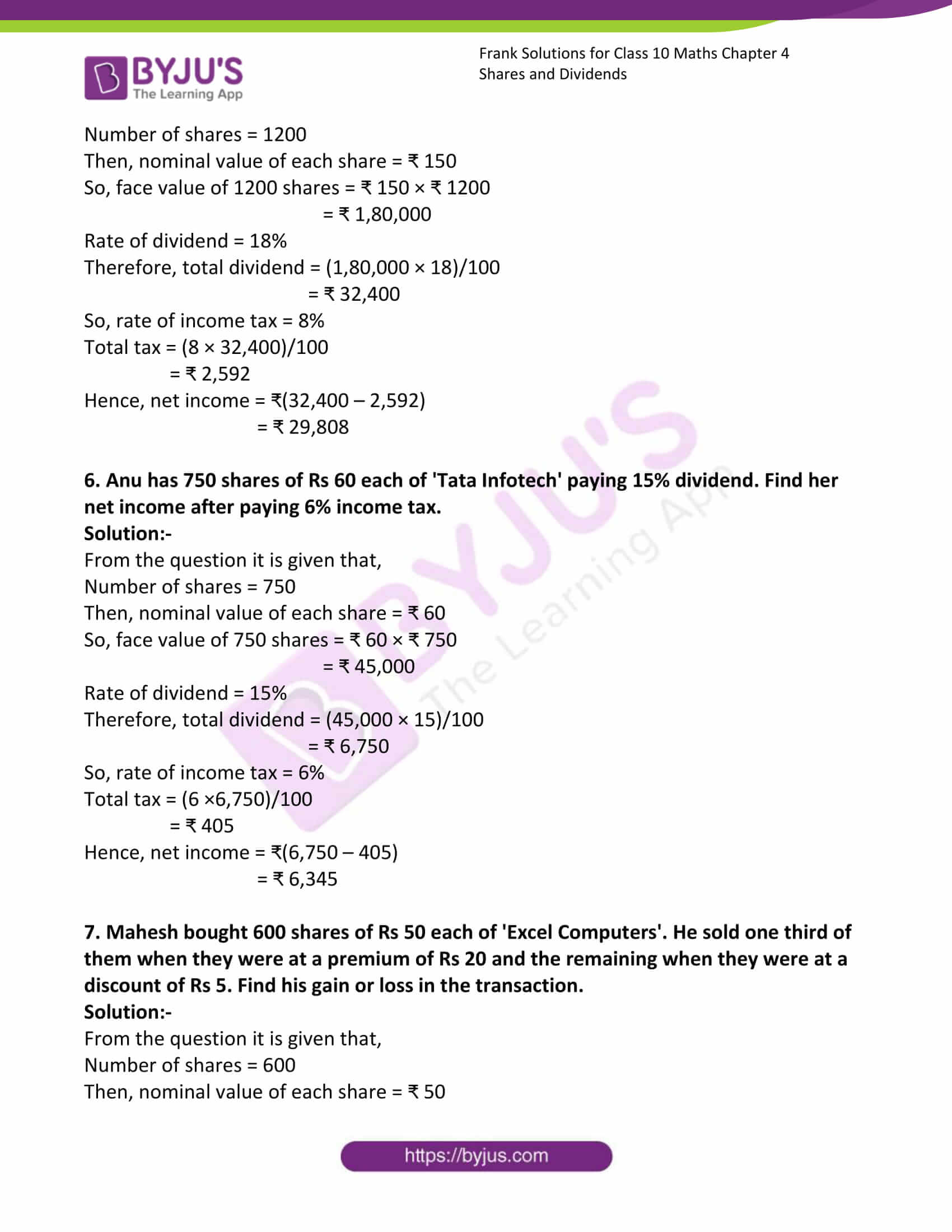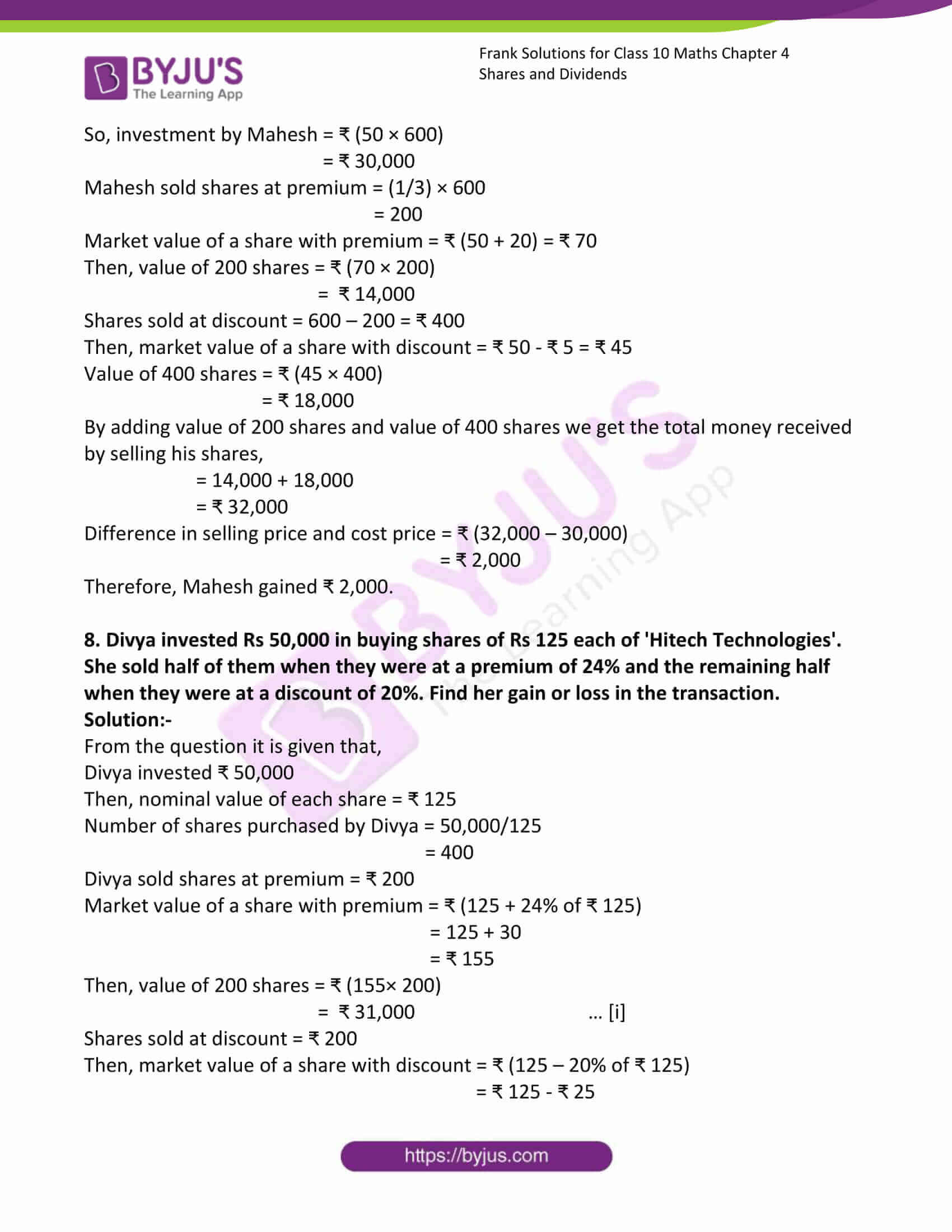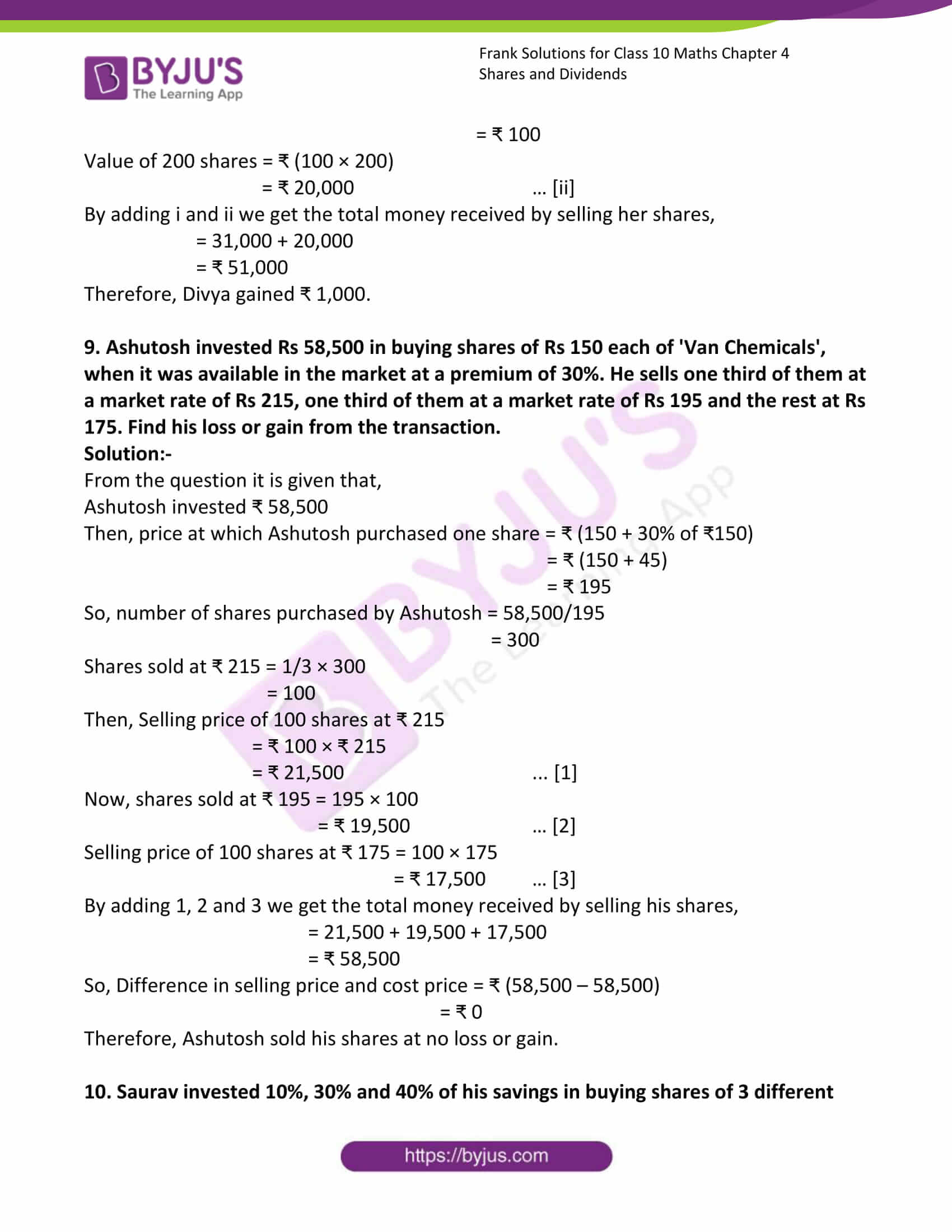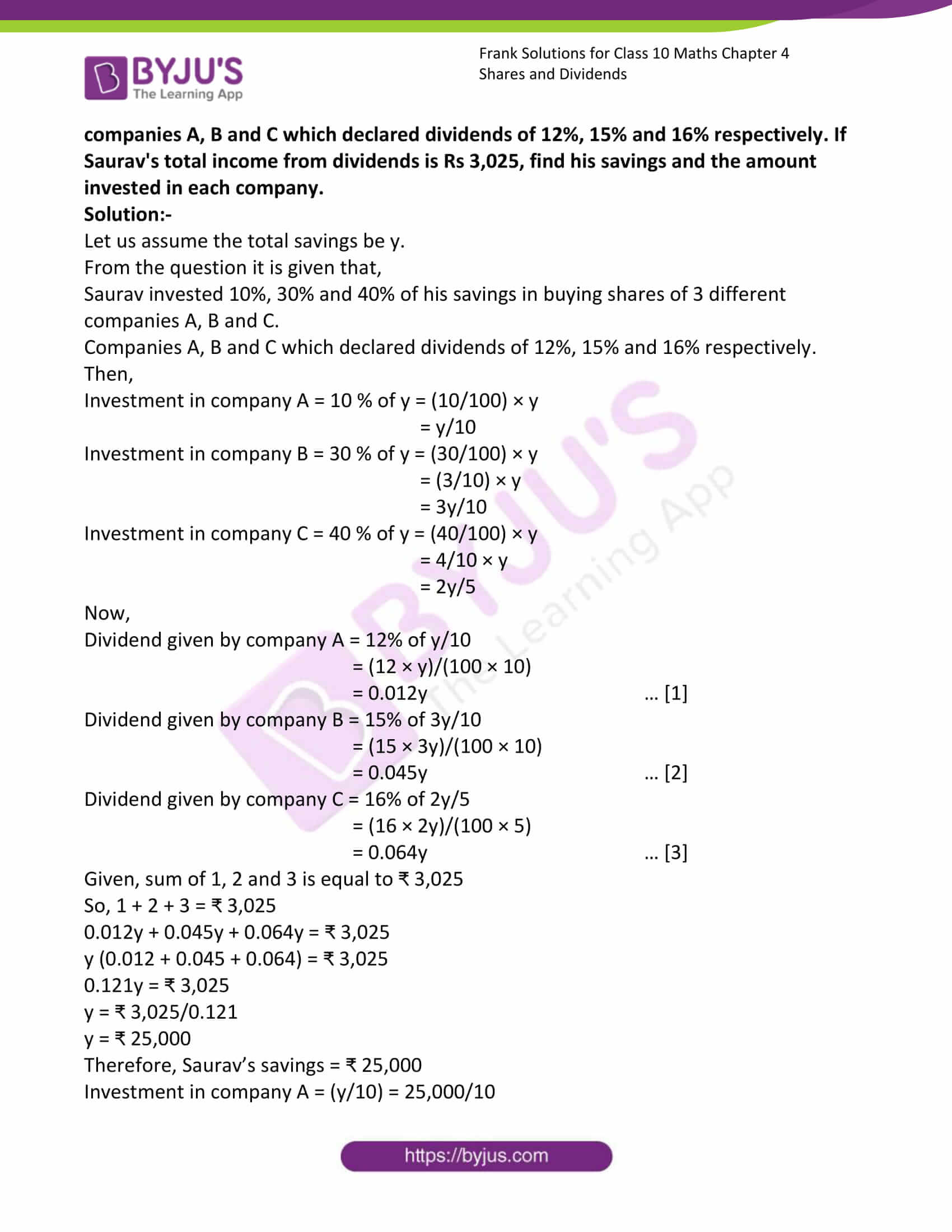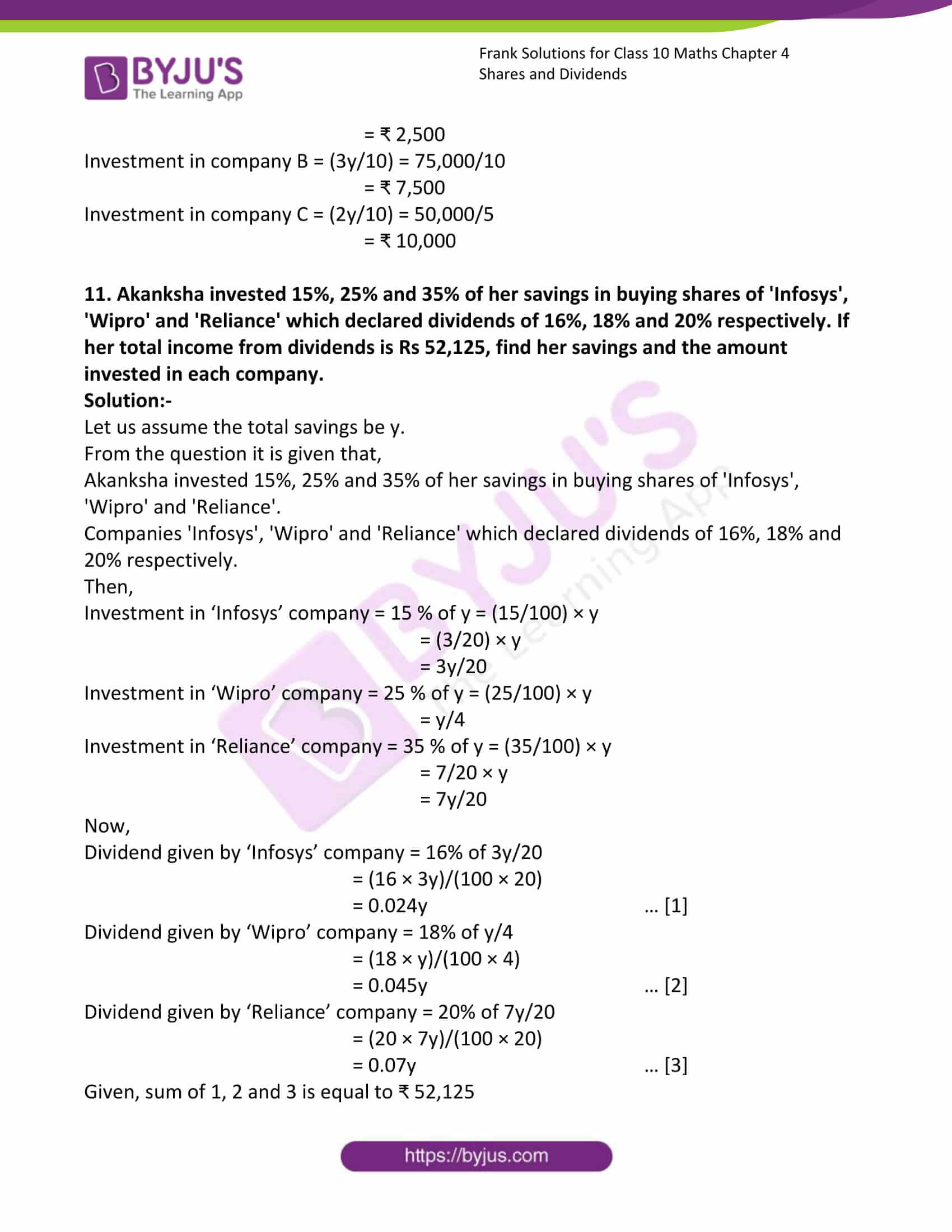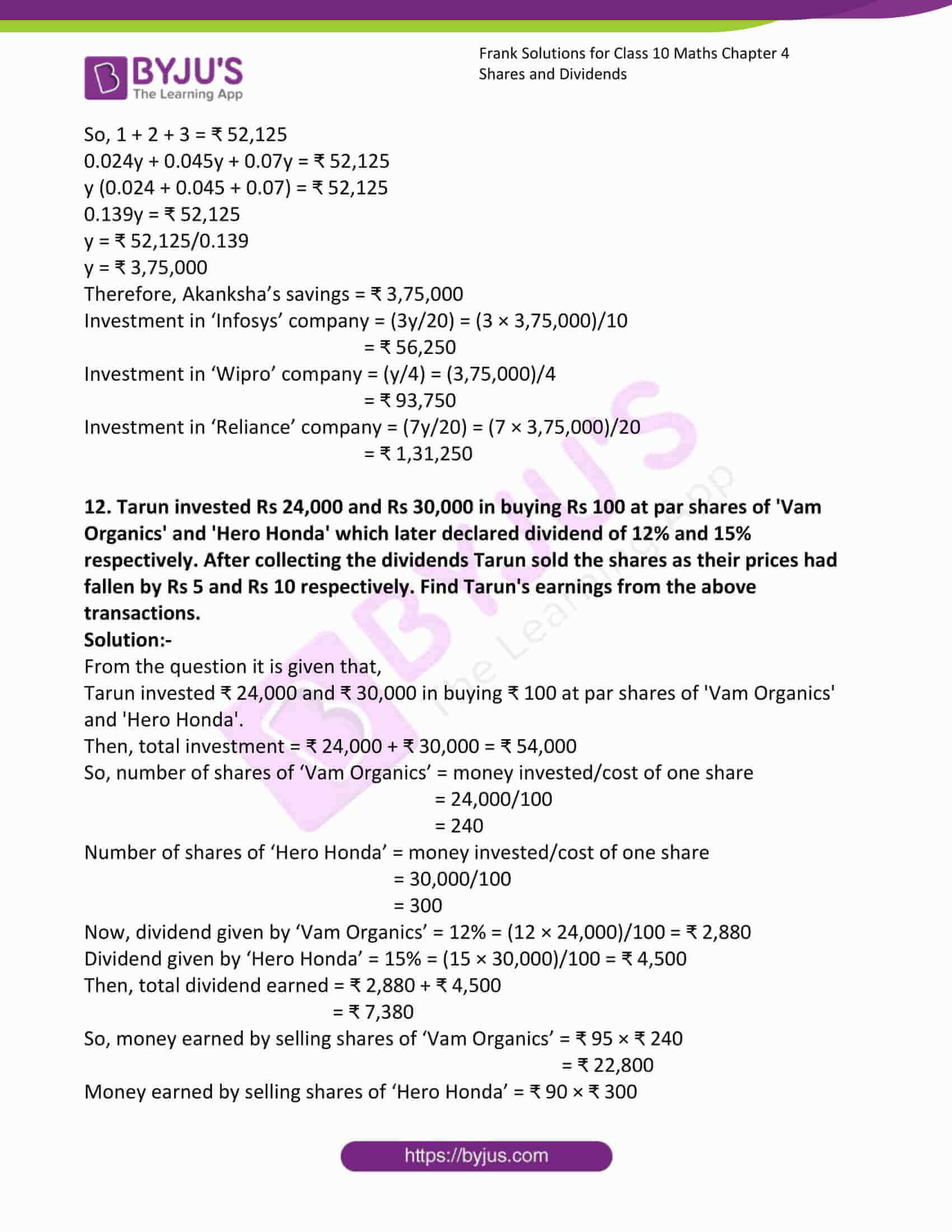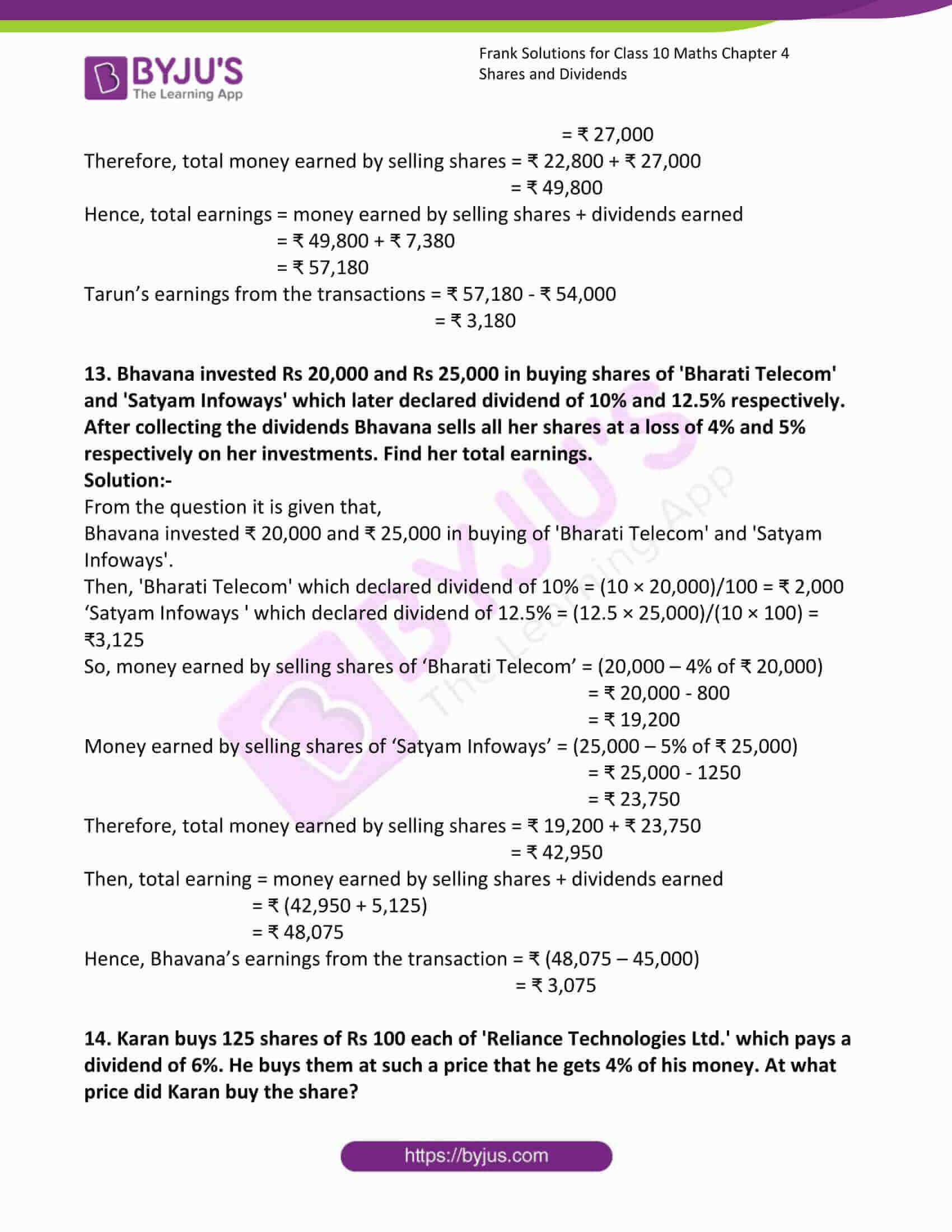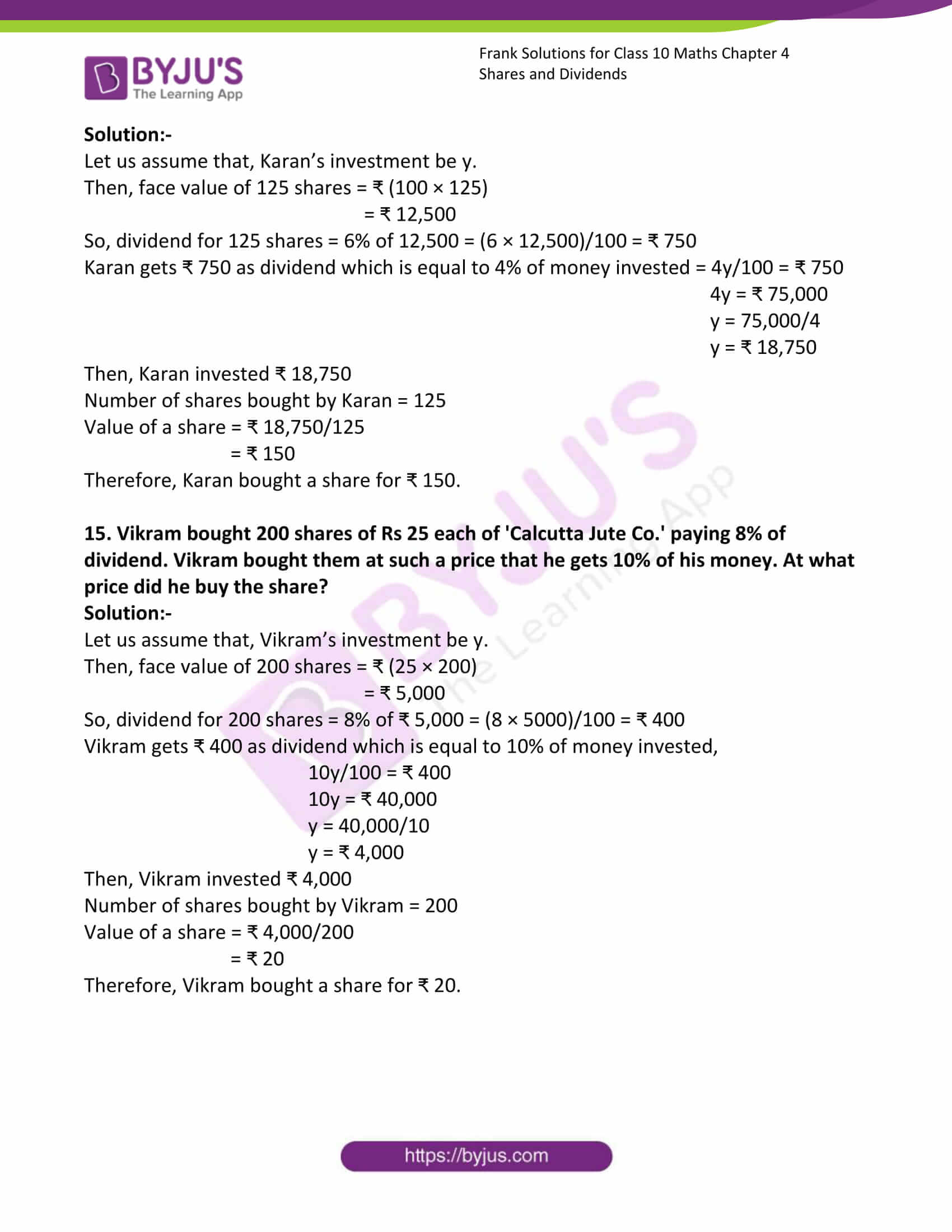### Access Answers to Frank Solutions for Class 10 Maths Chapter 4 Shares and Dividends

1. Calculate the investment required to buy:
(a) 500 shares of Rs 75 each at a premium of Rs 17.

Solution:-

From the question, it is given that,

The number of shares 500

Then, shares of ₹ 75 each at a premium of ₹ 17 = 75 + 17 = ₹ 92

So, the investment required to buy 500 shares = 92 × 500

= ₹ 46,000

(b) 315 shares of Rs 60 each at a premium of Rs 12.

Solution:-

From the question, it is given that,

The number of shares 315

Then, shares of ₹ 60 each at a premium of ₹ 12 = 60 + 12 = ₹ 72

So, the investment required to buy 315 shares = 72 × 315

= ₹ 22,680

(c) 600 shares of Rs 25 each at a discount of Rs 3.

Solution:-

From the question, it is given that,

The number of shares 600

Then, shares of ₹ 25 each at a discount of ₹ 3 = 25 – 3 = ₹ 22

So, the investment required to buy 600 shares = 22 × 600

= ₹ 13,200

(d) 425 shares of Rs 10 each at a discount of Rs 1.50.

Solution:-

From the question, it is given that,

The number of shares 425

Then, shares of ₹ 10 each at a discount of ₹ 1.50 = 10 – 1.50 = ₹ 8.50

So, the investment required to buy 600 shares = 8.50 × 425

= ₹ 3,612.50

(e) 250 shares of Rs 20 each at par.

Solution:-

From the question, it is given that,

The number of shares 250

Then, shares of ₹ 20 each at par

So, the investment required to buy 250 shares = 20 × 250

= ₹ 5,000

(f) 150 shares of Rs 100 each at a premium of 12%.

Solution:-

From the question, it is given that,

The number of shares 150

Then, shares of ₹ 100 each at a premium of 12% = (100 + 12% of ₹ 100)

= 100 + ((12/100) × 100)

= 100 + 12

= ₹ 112

So, the investment required to buy 150 shares = 112 × 150

= ₹ 16,800

(g) 220 shares of Rs 75 each at a premium of 15%.

Solution:-

From the question, it is given that,

The number of shares 220

Then, shares of ₹ 75 each at a premium of 15% = (75 + 15% of ₹ 75)

= 75 + ((15/100) × 75)

= 75 + 11.25

= ₹ 86.25

So, the investment required to buy 220 shares = 86.25 × 220

= ₹ 18,975

(h) 340 shares of Rs 125 each at a discount of 20%.

Solution:-

From the question, it is given that,

The number of shares 340

Then, shares of ₹ 125 each at a discount of 20% = (125 – 20% of ₹ 125)

= 125 – ((20/100) × 125)

= 125 – 25

= ₹ 100

So, the investment required to buy 220 shares = 340 × 100

= ₹ 34,000

(i) 750 shares of Rs 100 each at a discount of 24%.

Solution:-

From the question, it is given that,

The number of shares 750

Then, shares of ₹ 100 each at a discount of 24% = (100 – 24% of ₹ 100)

= 100 – ((24/100) × 100)

= 100 – 24

= ₹ 76

So, the investment required to buy 750 shares = 750 × 76

= ₹ 57,000

(j) 116 shares of Rs 125 each at par.

Solution:-

From the question, it is given that,

The number of shares 116

Then, shares of ₹ 125 each at par

So, the investment required to buy 116 shares = 125 × 116

= ₹ 14,500

2. Calculate the annual income of the following:
(a) 180 shares of Rs 50 each paying a 12% dividend.

Solution:-

From the question, it is given that,

The number of shares 180

Then, shares of ₹ 50,

Therefore, total investment = ₹ (50 × 180) = ₹ 9,000

Dividend = 12%

So, the annual Income = (12 × 9,000)/100

= ₹ 1,080

(b) 424 shares of Rs 125 each paying 8% dividend.

Solution:-

From the question, it is given that,

The number of shares 424

Then, shares of ₹ 125,

Therefore, total investment = ₹ (125 × 424) = ₹ 53,000

Dividend = 8%

So, the annual Income = (8 × 53,000)/100

= ₹ 4,240

(c) 60 shares of Rs 100 each available at Rs 75 and paying 5% dividend.

Solution:-

From the question, it is given that,

The number of shares 60

Then, shares of ₹ 100,

Therefore, total investment = ₹ (100 × 60) = ₹ 6,000

Dividend = 5%

So, the annual Income = (5 × 6,000)/100

= ₹ 300

(d) 120 shares of Rs 50 each available at Rs 62 and paying 13% dividend.

Solution:-

From the question, it is given that,

The number of shares 120

Then, shares of ₹ 50,

Therefore, total investment = ₹ (50 × 120) = ₹ 6,000

Dividend = 13%

So, the annual Income = (13 × 6,000)/100

= ₹ 780

3. Calculate the percentage income in the following investments:
(a) Rs 7,225 paying 12% when a Rs 100 share is available at 15% discount.

Solution:-

From the question, it is given that,

The nominal value of each share = ₹ 100 is available at 15% discount

Therefore, Market value = ₹ (100 – 15 % of ₹ 100)

= ₹ 100 – ₹ 15

= ₹ 85

Then, the number of shares purchased = 7,225/85

= ₹ 85

So, the face value of 85 shares = ₹ 100 × 85

= ₹ 8,500

Given, dividend = 12%

Annual income = (12 × 8,500)/100

= ₹ 1,020

Therefore, percentage income = (1,020 × 100)/7,225

= 14.12%

(b) Rs 7,168 paying 15% when Rs 80 share is available at 40% premium.

Solution:-

From the question, it is given that,

The nominal value of each share = ₹ 80 is available at 40% premium

Therefore, Market value = ₹ (80 + 40 % of ₹ 80)

= ₹ 80 + ₹ 32

= ₹ 112

Then, the number of shares purchased = 7,168/112

= ₹ 64

So, the face value of 64 shares = ₹ 80 × 64

= ₹ 5,120

Given, dividend = 15%

Annual income = (15 × 5,120)/100

= ₹ 768

Therefore, percentage income = (768 × 100)/7,168

= 10.71%

(c) Rs 36,250 in a Rs 125 share, paying 8% and available at a premium of Rs 20.

Solution:-

From the question, it is given that,

The nominal value of each share = ₹ 125 is available at 8% premium

Therefore, Market value = ₹ (125 + ₹ 20)

= ₹ 145

Then, the number of shares purchased = 36,250/145

= ₹ 250

So, the face value of 250 shares = ₹ 125 × 250

= ₹ 31,250

Given, dividend = 8%

Annual income = (8 × 31,250)/100

= ₹ 2,500

Therefore, percentage income = (2,500 × 100)/36,250

= 6.9%

(d) Rs 12,375 in a Rs 75 share paying 4% and available at a discount of Rs 20.

Solution:-

From the question, it is given that,

The nominal value of each share = ₹ 75 is available at discount

Therefore, Market value = (₹ 75 – ₹ 20)

= ₹ 55

Then, the number of shares purchased = 12,375/55

= ₹ 255

So, face value of 225 shares = ₹ 75 × ₹ 225

= ₹ 16,875

Given, dividend = 4%

Annual income = (4 × 16,875)/100

= ₹ 675

Therefore, percentage income = (675 × 100)/12,375

= 5.45%

4. Rani has 500 shares of Rs 125 each of a company paying 12% dividend. Find her net income after paying 5% income tax.

Solution:-

From the question, it is given that,

Number of shares = 500

Then, the nominal value of each share = ₹ 125

So, face value of 500 shares = ₹ 125 × ₹ 500

= ₹ 62,500

Rate of dividend = 12%

Therefore, total dividend = (62,500 × 12)/100

= ₹ 7,500

So, the rate of income tax = 5%

Total tax = (5 × 7500)/100

= ₹ 375

Hence, net income = ₹(7,500 – 375)

= ₹ 7,125

5. Yash has 1200 shares of Rs 150 each of ‘Honeywell Corporation’ paying 18% dividend. Find his net income after paying.

Solution:-

From the question, it is given that,

Number of shares = 1200

Then, the nominal value of each share = ₹ 150

So, face value of 1200 shares = ₹ 150 × ₹ 1200

= ₹ 1,80,000

Rate of dividend = 18%

Therefore, total dividend = (1,80,000 × 18)/100

= ₹ 32,400

So, the rate of income tax = 8%

Total tax = (8 × 32,400)/100

= ₹ 2,592

Hence, net income = ₹(32,400 – 2,592)

= ₹ 29,808

6. Anu has 750 shares of Rs 60 each of ‘Tata Infotech’ paying 15% dividend. Find her net income after paying 6% income tax.

Solution:-

From the question, it is given that,

Number of shares = 750

Then, the nominal value of each share = ₹ 60

So, face value of 750 shares = ₹ 60 × ₹ 750

= ₹ 45,000

Rate of dividend = 15%

Therefore, total dividend = (45,000 × 15)/100

= ₹ 6,750

So, rate of income tax = 6%

Total tax = (6 ×6,750)/100

= ₹ 405

Hence, net income = ₹(6,750 – 405)

= ₹ 6,345

7. Mahesh bought 600 shares of Rs 50 each of ‘Excel Computers’. He sold one-third of them when they were at a premium of Rs 20 and the remaining when they were at a discount of Rs 5. Find his gain or loss in the transaction.

Solution:-

From the question, it is given that,

Number of shares = 600

Then, the nominal value of each share = ₹ 50

So, investment by Mahesh = ₹ (50 × 600)

= ₹ 30,000

Mahesh sold shares at premium = (1/3) × 600

= 200

Market value of a share with premium = ₹ (50 + 20) = ₹ 70

Then, value of 200 shares = ₹ (70 × 200)

= ₹ 14,000

Shares sold at discount = 600 – 200 = ₹ 400

Then, market value of a share with discount = ₹ 50 – ₹ 5 = ₹ 45

Value of 400 shares = ₹ (45 × 400)

= ₹ 18,000

By adding the value of 200 shares and the value of 400 shares, we get the total money received by selling his shares,

= 14,000 + 18,000

= ₹ 32,000

Difference in selling price and cost price = ₹ (32,000 – 30,000)

= ₹ 2,000

Therefore, Mahesh gained ₹ 2,000.

8. Divya invested Rs 50,000 in buying shares of Rs 125 each of ‘Hitech Technologies’. She sold half of them when they were at a premium of 24% and the remaining half when they were at a discount of 20%. Find her gain or loss in the transaction.

Solution:-

From the question, it is given that,

Divya invested ₹ 50,000

Then, the nominal value of each share = ₹ 125

Number of shares purchased by Divya = 50,000/125

= 400

Divya sold shares at premium = ₹ 200

Market value of a share with premium = ₹ (125 + 24% of ₹ 125)

= 125 + 30

= ₹ 155

Then, value of 200 shares = ₹ (155× 200)

= ₹ 31,000 … [i]

Shares sold at discount = ₹ 200

Then, market value of a share with discount = ₹ (125 – 20% of ₹ 125)

= ₹ 125 – ₹ 25

= ₹ 100

Value of 200 shares = ₹ (100 × 200)

= ₹ 20,000 … [ii]

By adding i and ii we get the total money received by selling her shares,

= 31,000 + 20,000

= ₹ 51,000

Therefore, Divya gained ₹ 1,000.

9. Ashutosh invested Rs 58,500 in buying shares of Rs 150 each of ‘Van Chemicals’, when it was available in the market at a premium of 30%. He sells one-third of them at a market rate of Rs 215, one-third of them at a market rate of Rs 195 and the rest at Rs 175. Find his loss or gain from the transaction.

Solution:-

From the question, it is given that,

Ashutosh invested ₹ 58,500

Then, price at which Ashutosh purchased one share = ₹ (150 + 30% of ₹150)

= ₹ (150 + 45)

= ₹ 195

So, the number of shares purchased by Ashutosh = 58,500/195

= 300

Shares sold at ₹ 215 = 1/3 × 300

= 100

Then, the selling price of 100 shares at ₹ 215

= ₹ 100 × ₹ 215

= ₹ 21,500 … 

Now, shares sold at ₹ 195 = 195 × 100

= ₹ 19,500 … 

Selling price of 100 shares at ₹ 175 = 100 × 175

= ₹ 17,500 … 

By adding 1, 2 and 3 we get the total money received by selling his shares,

= 21,500 + 19,500 + 17,500

= ₹ 58,500

So, the difference in selling price and cost price = ₹ (58,500 – 58,500)

= ₹ 0

Therefore, Ashutosh sold his shares at no loss or gain.

10. Saurav invested 10%, 30% and 40% of his savings in buying shares of 3 different companies, A, B and C, which declared dividends of 12%, 15% and 16%, respectively. If Saurav’s total income from dividends is Rs 3,025, find his savings and the amount invested in each company.

Solution:-

Let us assume the total savings be y.

From the question, it is given that,

Saurav invested 10%, 30% and 40% of his savings in buying shares of 3 different companies A, B and C.

Companies A, B and C declared dividends of 12%, 15% and 16%, respectively.

Then,

Investment in company A = 10 % of y = (10/100) × y

= y/10

Investment in company B = 30 % of y = (30/100) × y

= (3/10) × y

= 3y/10

Investment in company C = 40 % of y = (40/100) × y

= 4/10 × y

= 2y/5

Now,

Dividend given by company A = 12% of y/10

= (12 × y)/(100 × 10)

= 0.012y … 

Dividend given by company B = 15% of 3y/10

= (15 × 3y)/(100 × 10)

= 0.045y … 

Dividend given by company C = 16% of 2y/5

= (16 × 2y)/(100 × 5)

= 0.064y … 

Given, the sum of 1, 2 and 3 is equal to ₹ 3,025

So, 1 + 2 + 3 = ₹ 3,025

0.012y + 0.045y + 0.064y = ₹ 3,025

y (0.012 + 0.045 + 0.064) = ₹ 3,025

0.121y = ₹ 3,025

y = ₹ 3,025/0.121

y = ₹ 25,000

Therefore, Saurav’s savings = ₹ 25,000

Investment in company A = (y/10) = 25,000/10

= ₹ 2,500

Investment in company B = (3y/10) = 75,000/10

= ₹ 7,500

Investment in company C = (2y/10) = 50,000/5

= ₹ 10,000

11. Akanksha invested 15%, 25% and 35% of her savings in buying shares of ‘Infosys’, ‘Wipro’ and ‘Reliance’, which declared dividends of 16%, 18% and 20%, respectively. If her total income from dividends is Rs 52,125, find her savings and the amount invested in each company.

Solution:-

Let us assume the total savings be y.

From the question, it is given that,

Akanksha invested 15%, 25% and 35% of her savings in buying shares of ‘Infosys’, ‘Wipro’ and ‘Reliance’.

Companies ‘Infosys’, ‘Wipro’ and ‘Reliance’ declared dividends of 16%, 18% and 20%, respectively.

Then,

Investment in ‘Infosys’ company = 15 % of y = (15/100) × y

= (3/20) × y

= 3y/20

Investment in ‘Wipro’ company = 25 % of y = (25/100) × y

= y/4

Investment in ‘Reliance’ company = 35 % of y = (35/100) × y

= 7/20 × y

= 7y/20

Now,

Dividend given by ‘Infosys’ company = 16% of 3y/20

= (16 × 3y)/(100 × 20)

= 0.024y … 

Dividend given by ‘Wipro’ company = 18% of y/4

= (18 × y)/(100 × 4)

= 0.045y … 

Dividend given by ‘Reliance’ company = 20% of 7y/20

= (20 × 7y)/(100 × 20)

= 0.07y … 

Given, the sum of 1, 2 and 3 is equal to ₹ 52,125

So, 1 + 2 + 3 = ₹ 52,125

0.024y + 0.045y + 0.07y = ₹ 52,125

y (0.024 + 0.045 + 0.07) = ₹ 52,125

0.139y = ₹ 52,125

y = ₹ 52,125/0.139

y = ₹ 3,75,000

Therefore, Akanksha’s savings = ₹ 3,75,000

Investment in ‘Infosys’ company = (3y/20) = (3 × 3,75,000)/10

= ₹ 56,250

Investment in ‘Wipro’ company = (y/4) = (3,75,000)/4

= ₹ 93,750

Investment in ‘Reliance’ company = (7y/20) = (7 × 3,75,000)/20

= ₹ 1,31,250

12. Tarun invested Rs 24,000 and Rs 30,000 in buying Rs 100 at par shares of ‘Vam Organics’ and ‘Hero Honda’ which later declared dividends of 12% and 15%, respectively. After collecting the dividends, Tarun sold the shares as their prices had fallen by Rs 5 and Rs 10, respectively. Find Tarun’s earnings from the above transactions.

Solution:-

From the question, it is given that,

Tarun invested ₹ 24,000 and ₹ 30,000 in buying ₹ 100 at par shares of ‘Vam Organics’ and ‘Hero Honda’.

Then, total investment = ₹ 24,000 + ₹ 30,000 = ₹ 54,000

So, the number of shares of ‘Vam Organics’ = money invested/cost of one share

= 24,000/100

= 240

Number of shares of ‘Hero Honda’ = money invested/cost of one share

= 30,000/100

= 300

Now, dividend given by ‘Vam Organics’ = 12% = (12 × 24,000)/100 = ₹ 2,880

Dividend given by ‘Hero Honda’ = 15% = (15 × 30,000)/100 = ₹ 4,500

Then, total dividend earned = ₹ 2,880 + ₹ 4,500

= ₹ 7,380

So, money earned by selling shares of ‘Vam Organics’ = ₹ 95 × ₹ 240

= ₹ 22,800

Money earned by selling shares of ‘Hero Honda’ = ₹ 90 × ₹ 300

= ₹ 27,000

Therefore, total money earned by selling shares = ₹ 22,800 + ₹ 27,000

= ₹ 49,800

Hence, total earnings = money earned by selling shares + dividends earned

= ₹ 49,800 + ₹ 7,380

= ₹ 57,180

Tarun’s earnings from the transactions = ₹ 57,180 – ₹ 54,000

= ₹ 3,180

13. Bhavana invested Rs 20,000 and Rs 25,000 in buying shares of ‘Bharati Telecom’ and ‘Satyam Infoways’ which later declared dividends of 10% and 12.5%, respectively. After collecting the dividends, Bhavana sells all her shares at a loss of 4% and 5%, respectively, on her investments. Find her total earnings.

Solution:-

From the question, it is given that,

Bhavana invested ₹ 20,000 and ₹ 25,000 in buying ‘Bharati Telecom’ and ‘Satyam Infoways’.

Then, ‘Bharati Telecom’ which declared a dividend of 10% = (10 × 20,000)/100 = ₹ 2,000

‘Satyam Infoways ‘ which declared dividend of 12.5% = (12.5 × 25,000)/(10 × 100) = ₹3,125

So, money earned by selling shares of ‘Bharati Telecom’ = (20,000 – 4% of ₹ 20,000)

= ₹ 20,000 – 800

= ₹ 19,200

Money earned by selling shares of ‘Satyam Infoways’ = (25,000 – 5% of ₹ 25,000)

= ₹ 25,000 – 1250

= ₹ 23,750

Therefore, total money earned by selling shares = ₹ 19,200 + ₹ 23,750

= ₹ 42,950

Then, total earning = money earned by selling shares + dividends earned

= ₹ (42,950 + 5,125)

= ₹ 48,075

Hence, Bhavana’s earnings from the transaction = ₹ (48,075 – 45,000)

= ₹ 3,075

14. Karan buys 125 shares of Rs 100 each of ‘Reliance Technologies Ltd.’, which pays a dividend of 6%. He buys them at such a price that he gets 4% of his money. At what price did Karan buy the share?

Solution:-

Let us assume that, Karan’s investment is y.

Then, face value of 125 shares = ₹ (100 × 125)

= ₹ 12,500

So, dividend for 125 shares = 6% of 12,500 = (6 × 12,500)/100 = ₹ 750

Karan gets ₹ 750 as dividend, which is equal to 4% of the money invested = 4y/100 = ₹ 750

4y = ₹ 75,000

y = 75,000/4

y = ₹ 18,750

Then, Karan invested ₹ 18,750

Number of shares bought by Karan = 125

Value of a share = ₹ 18,750/125

= ₹ 150

Therefore, Karan bought a share for ₹ 150.

15. Vikram bought 200 shares of Rs 25 each of ‘Calcutta Jute Co.’, paying 8% of the dividend. Vikram bought them at such a price that he gets 10% of his money. At what price did he buy the share?

Solution:-

Let us assume that, Vikram’s investment is y.

Then, face value of 200 shares = ₹ (25 × 200)

= ₹ 5,000

So, dividend for 200 shares = 8% of ₹ 5,000 = (8 × 5000)/100 = ₹ 400

Vikram gets ₹ 400 as dividend, which is equal to 10% of the money invested,

10y/100 = ₹ 400

10y = ₹ 40,000

y = 40,000/10

y = ₹ 4,000

Then, Vikram invested ₹ 4,000

Number of shares bought by Vikram = 200

Value of a share = ₹ 4,000/200

= ₹ 20

Therefore, Vikram bought a share for ₹ 20.